# 机器学习笔记(一)

### 先写出一个带有未知参数的数学式

$Function \ with \ Unknown \ Parameters$

$y = b + w x_1$

$x_1:$ 公式中已知的东西叫做feature

$w:$ 不知道的参数叫做weight（权重）

$b:$ 不知道的参数叫做bias （偏移）

### 根据训练集定义$Loss$

$Loss(b,w)$是一个函数，输入是$b,w$

Loss代表训练出来的函数对于训练集的拟合程度

$Loss: L = \frac{1}{N}\sum\limits_n{e_n} (e = |y - y’|)$ 每项$e$是$model$对于训练集的误差

### 最佳化

$w^{\star}, b^{\star} = arg \ \min\limits_{w,b}L$

#### $Gradient\ Descent$

• 随机选取一个初始点$w^0$
• 计算微分 $\frac{\partial L}{\partial w}|_{w= w^0}$
• 根据微分的正负选择向前或向后移动$\eta\frac{\partial L}{\partial w}|_{w= w^0}$, $\eta$学习速率，自己设置
• 迭代的更新$w$值

## $model$ 的限制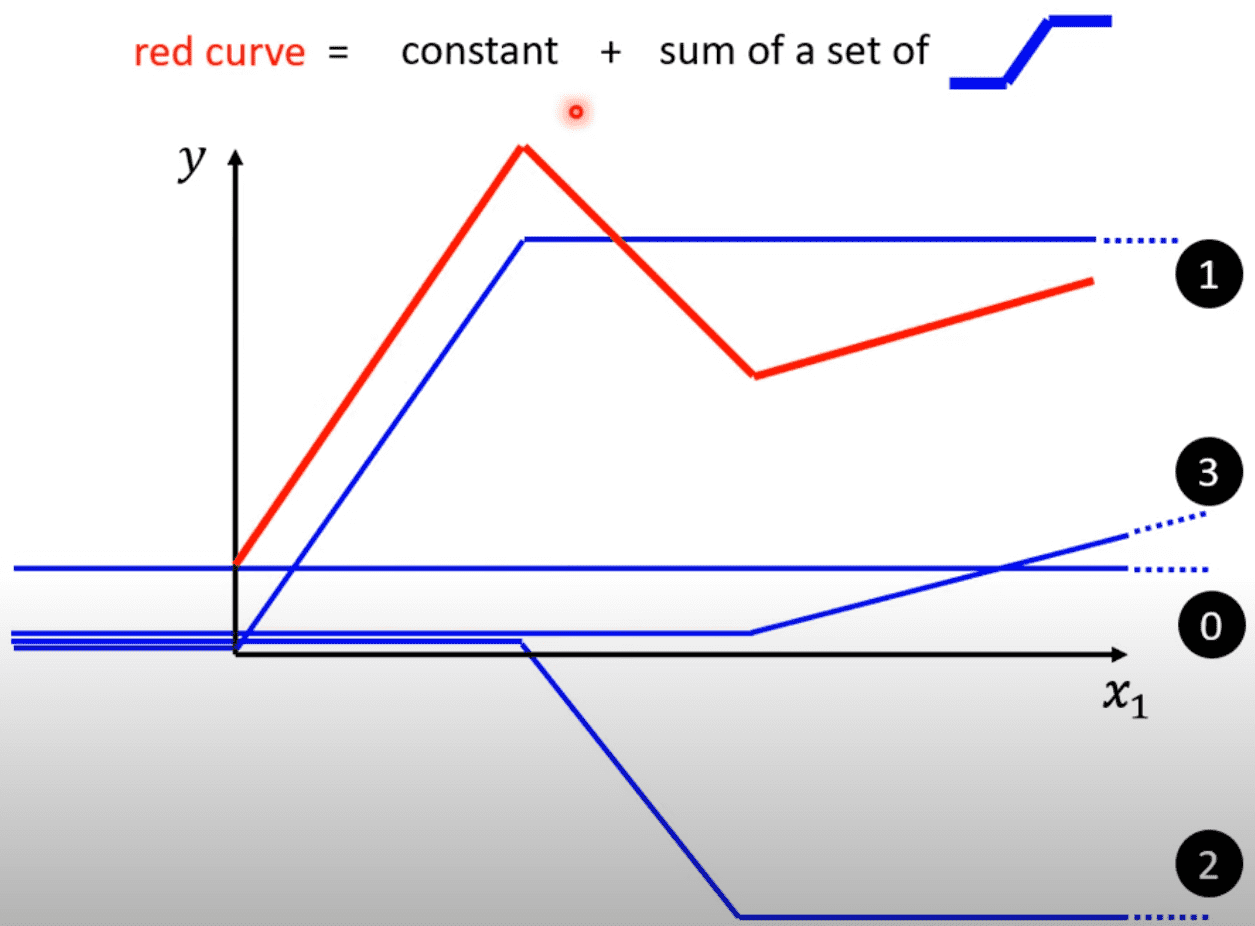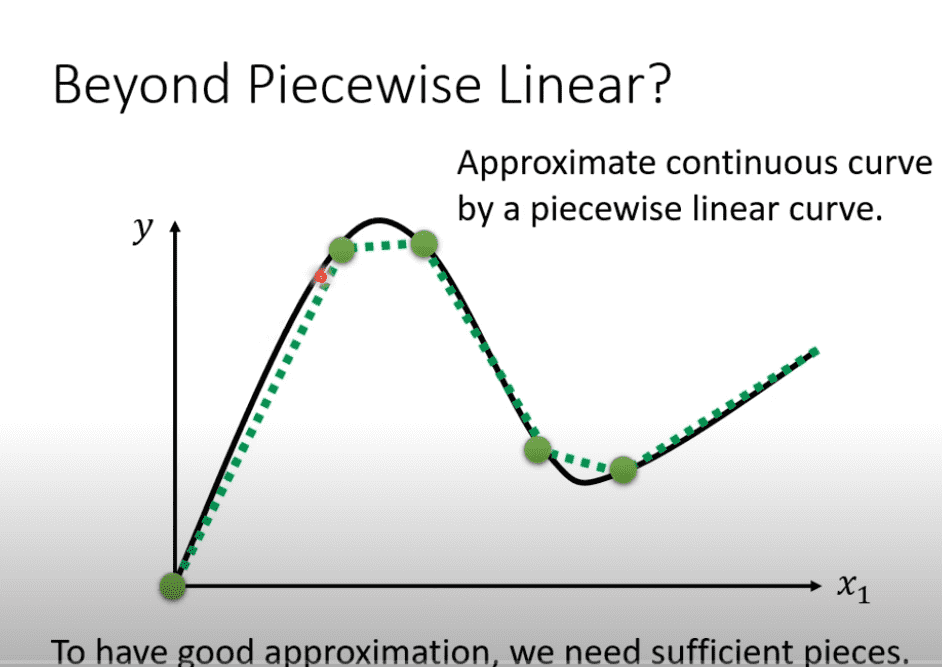### 如何来表达蓝色线段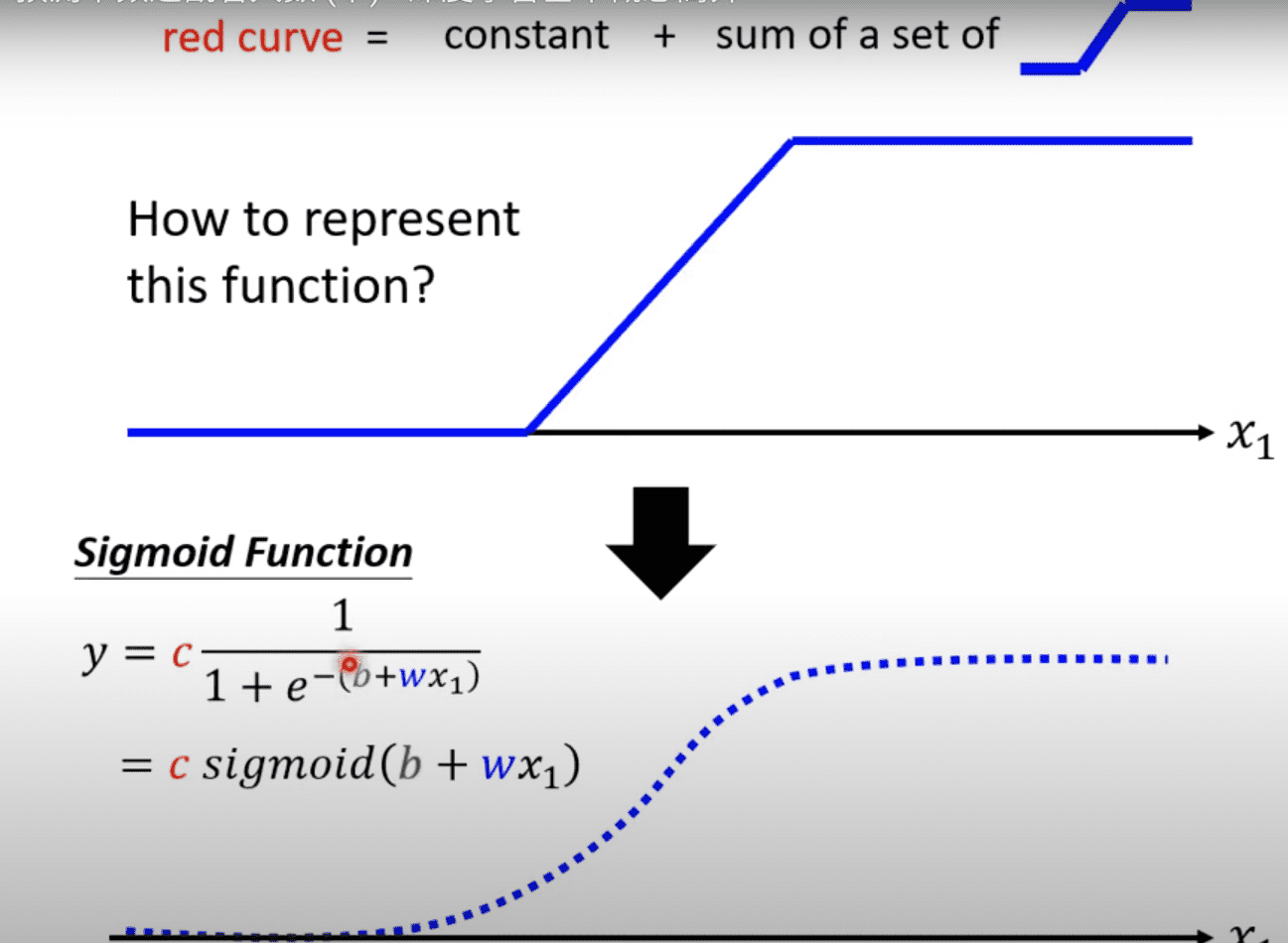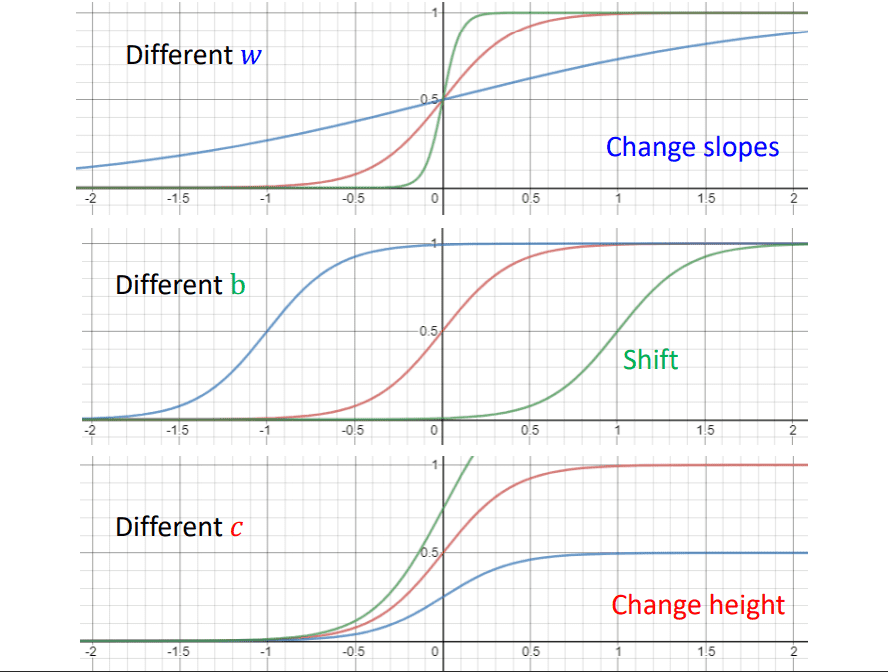### 线性代数表达

$\sum\limits_{i}c_i\ sigmoid(b_i + w_ix_1)$可以表示为

$r = b + Wx$

#### $Loss$ 使用$\theta$参数

$Loss$现在使用写成$L(\theta)$

#### 迭代最好的$model$

$\theta^* = arg \ \min\limits_{\theta}L$

$g = \begin{bmatrix} \frac{\partial L}{\partial \theta_1}|_{\theta = \theta^0} \\ \frac{\partial L}{\partial \theta_2}|_{\theta = \theta^0} \\ \frac{\partial L}{\partial \theta_3}|_{\theta = \theta^0} \\ \dots \end{bmatrix}= \nabla L (\theta ^0)$

$\begin{bmatrix} \theta_1^1 \\ \theta_2^1\\ \dots \end{bmatrix} \longleftarrow \begin{bmatrix} \theta_1^0 \\ \theta_2^0\\ \dots \end{bmatrix} - \begin{bmatrix} \eta \frac{\partial L}{\partial \theta_1}|_{\theta = \theta^0} \\ \eta \frac{\partial L}{\partial \theta_2}|_{\theta = \theta^0} \\ \dots \end{bmatrix}$

$\theta^1 \longleftarrow \theta^0 - \eta g$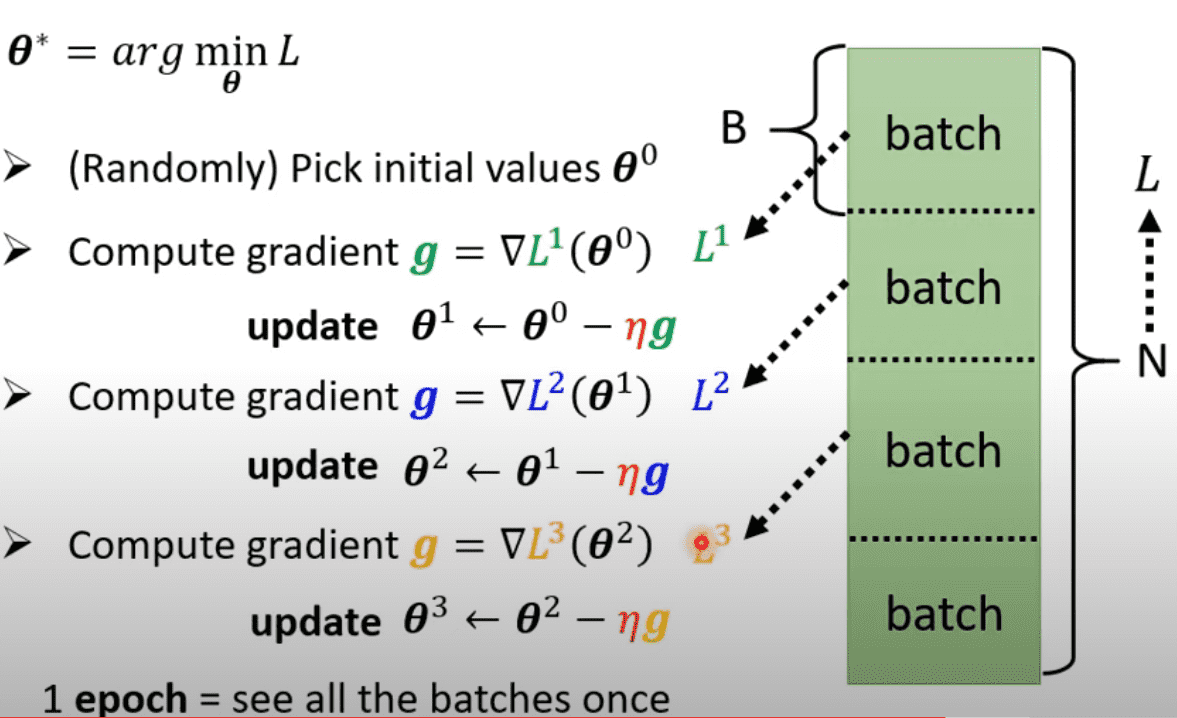### 从 $sigmoid$到$RELU$

$y = b + \sum\limits_i c_i \ sigmoid(b_i + \sum\limits_jw_{ij}x_j)$

$y = b + \sum\limits_{2i}c_i max(0, b_i+\sum\limits_jw_{ij}x_j)$

$sigmoid$ 和 $RELU$ 统称为$activation\ function$, $RELU$更好一些

目录